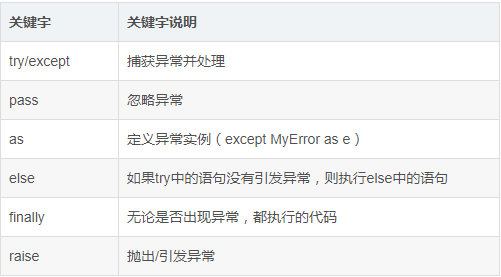# 异常捕获## 1 捕获所有异常

``````try:
<语句>
except:
print('异常说明')
``````

## 2 捕获指定异常

``````try:
<语句>
except <异常名>:
print('异常说明')
``````

``````try:
<语句>
except Exception:
print('异常说明')
``````

``````try:
f = open("file-not-exists", "r")
except IOError as e:
print("open exception: %s: %s" %(e.errno, e.strerror))
``````

## 3 捕获多个异常

``````try:
<语句>
except (<异常名1>, <异常名2>, ...):
print('异常说明')
``````

``````try:
<语句>

except <异常名1>:

print('异常说明1')

except <异常名2>:

print('异常说明2')

except <异常名3>:

print('异常说明3')
``````

## 4 异常中的 else

``````try:
<语句>
except <异常名1>:
print('异常说明1')
except <异常名2>:
print('异常说明2')
else:
<语句> # try语句中没有异常则执行此段代码
``````

## 5 异常中的 finally

try…finally…语句无论是否发生异常都将会执行最后的代码。

``````try:
<语句>
finally:
<语句>
``````

``````str1 = 'hello world'
try:
int(str1)
except IndexError as e:
print(e)
except KeyError as e:
print(e)
except ValueError as e:
print(e)
else:
print('try内没有异常')
finally:
print('无论异常与否,都会执行我')
``````

## 6 raise 主动触发异常

raise [Exception [, args [, traceback]]]

``````def not_zero(num):
try:
if num == 0:
raise ValueError('参数错误')
return num
except Exception as e:
print(e)
not_zero(0)
``````

## 7 采用 traceback 模块查看异常 (RPA 已默认封装)

``````try:
block
except:
traceback.print_exc()
``````

``````try:
1/0
except Exception as e:
print(e)
``````

``````import traceback

try:
1/0
except Exception as e:
traceback.print_exc()
``````

Traceback (most recent call last):
File “E:/PycharmProjects/ProxyPool-master/proxypool/test.py”, line 4, in
1/0
ZeroDivisionError: division by zero

traceback.print_exc(file=open(‘tb.txt’,‘w+’))